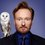Prove that $m$ is an integer bigger than 11 then there always exist 2 different composite integers $x$ and $y$ such that $m=x+y$.Note by Christian Warjri
4 years ago

This discussion board is a place to discuss our Daily Challenges and the math and science related to those challenges. Explanations are more than just a solution — they should explain the steps and thinking strategies that you used to obtain the solution. Comments should further the discussion of math and science.

When posting on Brilliant:

• Use the emojis to react to an explanation, whether you're congratulating a job well done , or just really confused .
• Ask specific questions about the challenge or the steps in somebody's explanation. Well-posed questions can add a lot to the discussion, but posting "I don't understand!" doesn't help anyone.
• Try to contribute something new to the discussion, whether it is an extension, generalization or other idea related to the challenge.

MarkdownAppears as
*italics* or _italics_ italics
**bold** or __bold__ bold
- bulleted- list
• bulleted
• list
1. numbered2. list
1. numbered
2. list
Note: you must add a full line of space before and after lists for them to show up correctly
paragraph 1paragraph 2

paragraph 1

paragraph 2

[example link](https://brilliant.org)example link
> This is a quote
This is a quote
    # I indented these lines
# 4 spaces, and now they show
# up as a code block.

print "hello world"
# I indented these lines
# 4 spaces, and now they show
# up as a code block.

print "hello world"
MathAppears as
Remember to wrap math in $$ ... $$ or $ ... $ to ensure proper formatting.
2 \times 3 $2 \times 3$
2^{34} $2^{34}$
a_{i-1} $a_{i-1}$
\frac{2}{3} $\frac{2}{3}$
\sqrt{2} $\sqrt{2}$
\sum_{i=1}^3 $\sum_{i=1}^3$
\sin \theta $\sin \theta$
\boxed{123} $\boxed{123}$

Sort by:

If we take $m \equiv 0 \pmod4$, we can take $x=8$ and y as the required multiple of 4. Note that 16 has to written as 10+6.

If we take $m \equiv 1 \pmod4$, we can take $x=9$ and y as the required multiple of 4.

If we take $m \equiv 2 \pmod4$, we can take $x=10$ and y as the required multiple of 4.

If we take $m \equiv 3 \pmod4$, we can take $x=15$ and y as the required multiple of 4.

Great!

Depending on the intent of the question, you might be missing one small case.
Note: Most people do not consider 0 a composite number.

Staff - 4 years ago

Oh right! 15 can be written as $6+9$.

Will you please help me out in solving a question as There is a right angle isosceles triangle ABC 90 degree angle at B and AC is hypotenuse. There are two points D, E in between AC such that AD:DE:EC=3:5:4 then prove that angle DBE=45degree

- 2 years, 10 months ago

$m>11 \\ m = 11 + a ; ~ a \in Q\\ a = \pm n, ~ n \in Q \\ m = x + y, ~x = 11, y = \pm n$

- 4 years ago

Can you explain what you are trying to do? I have several concerns:

1. 11 isn't composite.
2. For $m = 13$, $13 = 11 + 2$, 2 isn't composite either.

Staff - 4 years ago

Ahh, is this sum that tough? I am doomed.

- 4 years ago

Nope, I am pointing out that the solution makes no sense, and does not answer the problem at all.

It's actually a pretty easy problem, just give it a try. What have you tried? Where did you get stuck?

Staff - 4 years ago

oh pretty sure i dont notice the condition it needs to be a composite number

- 4 years ago

13 = 9 + 4

- 2 years, 11 months ago